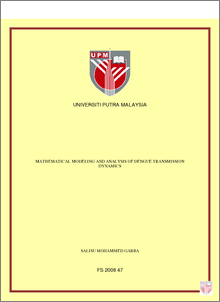# Mathematical Modeling and Analysis of Dengue Transmission Dynamics

## Citation

Mohammed Garba, Salisu (2008) Mathematical Modeling and Analysis of Dengue Transmission Dynamics. PhD thesis, Universiti Putra Malaysia.

## Abstract

The work in this thesis is based on the design and analysis of suitable compartmental deterministic models for the transmission dynamics of dengue fever in a population. A basic dengue model which allows transmission by exposed humans and mosquitoes is developed and rigorously analysed. The model, consisting of seven mutually-exclusive compartments representing the human and vector dynamics, has a locally-asymptotically stable (LAS) diseasefree equilibrium (DFE) whenever a certain epidemiological threshold, known as the basic reproduction number (Ro) is less than unity. Further, the model exhibits the phenomenon of backward bifurcation, where the stable DFE co-exists with a stable endemic equilibrium. The epidemiological consequence of this phenomenon is that the classical epidemiological requirement of making Ro less than unity is no longer sufficient, although necessary, for effectively controlling the spread of dengue in a community. The model is extended to incorporate an imperfect vaccine against the strain of dengue. In both the original and the extended models, it is shown, using Lyapunov function theory and LaSalle Invariance Principle, that the backward bifurcation phenomenon can be removed by substituting the associated standard incidence function with a mass action incidence. In other words, in addition to establishing the presence of backward bifurcation in models of dengue transmission, this study shows that the use of standard incidence in modelling dengue disease causes the backward bifurcation phenomenon of dengue disease. The model is extended to include the dynamics of two strains of dengue disease. The extended model has a locally-asymptotically stable, disease-free equilibrium (DFE) whenever the maximum of the associated reproduction numbers of the two strains (denoted by Ro) is less than unity. It is also shown, using a Lyapunov function and LaSalle Invariance Principle, that the DFE of the model, in the absence of dengue-induced mortality, is globally-asymptotically stable whenever Ro<1. The two strains co-exist if the reproduction number of each strain exceeds unity (and are different). For the case when the two reproduction numbers exceed unity but are equal, a continuum of co-existence equilibria exists. The impact of cross-immunity is explored for the case when Ro >1. It is shown that the model can have infinitely many co-existence equilibria if infection with one strain confers complete immunity against the other strain. However, if infection with one strain has no effect on susceptibility to the other strain, the model can have a unique co-existence equilibrium. It is shown that cross-immunity could lead to disease elimination, competitive exclusion or co-existence of the strains. Further, the effect of seasonality on dengue transmission dynamics is explored using numerical simulations. It is shown that the oscillation pattern differs between the strains, both in their subharmonic periods and the relative phase of cycles, depending on the degree of the cross-immunity between the strains. Finally, a deterministic model for monitoring the impact of treatment and vector control strategy on the transmission dynamics of dengue in the human and vector populations is formulated. In addition to having a locally-asymptotically stable disease-free equilibrium (DFE) whenever Ro is less than unity, it is shown, using a Lyapunov function and LaSalle Invariance Principle, and using comparison theorem that the DFE of both treatment-free and treatment model, in the absence of dengue-induced mortality, is globally-asymptotically stable whenever Ro <1; each of the models has a unique endemic equilibrium whenever its reproduction number exceed unity. Numerical simulations shows that, the use of vector control strategies can result in the effective control of dengue in a community by reducing the population of susceptible and exposed mosquitoes.Preview
PDF
FS_2008_47a.pdfView Item